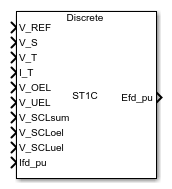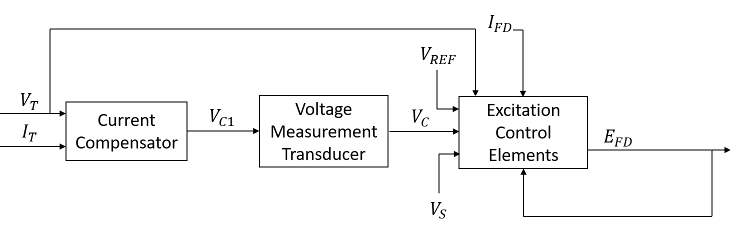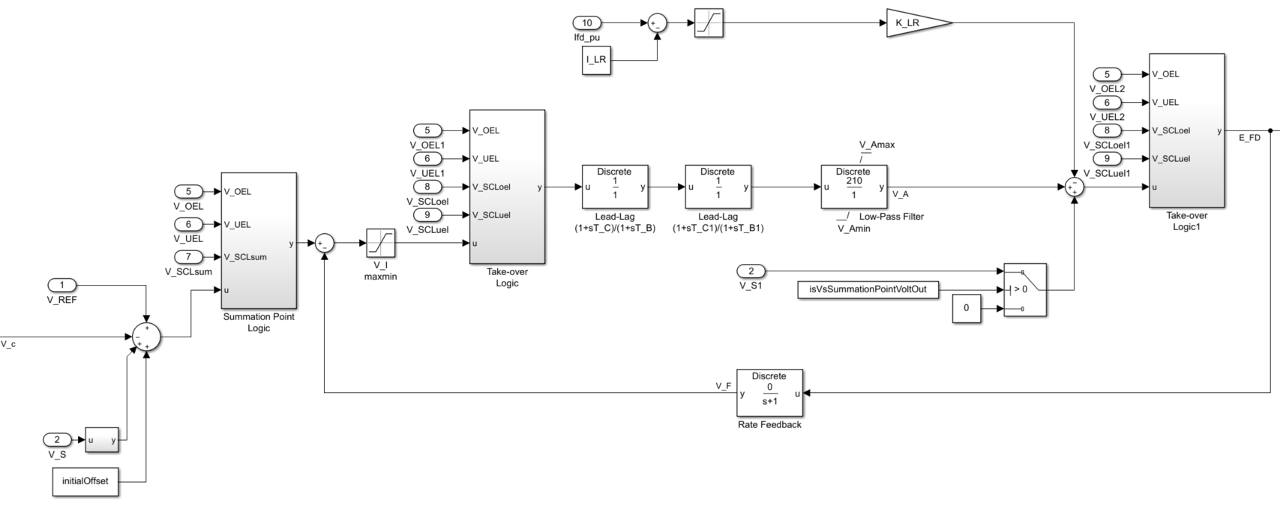# SM ST1C

Discrete-time or continuous-time synchronous machine ST1C static excitation system with an automatic voltage regulator

• Library:
• Simscape / Electrical / Control / SM Control

•## Description

The SM ST1C block implements a synchronous-machine-type ST1C static excitation system model in conformance with IEEE 421.5-2016.

Use this block to model the control and regulation of the field voltage of a synchronous machine.

You can switch between continuous and discrete implementations of the block by using the Sample time (-1 for inherited) parameter. To configure the integrator for continuous time, set the Sample time (-1 for inherited) property to `0`. To configure the integrator for discrete time, set the Sample time (-1 for inherited) property to a positive, nonzero value, or to `-1` to inherit the sample time from an upstream block.

The SM ST1C block comprises three major components:

• The Current Compensator modifies the measured terminal voltage as a function of the terminal current.

• The Voltage Measurement Transducer simulates the dynamics of a terminal voltage transducer using a low-pass filter.

• The Excitation Control Elements component compares the voltage transducer output with a terminal voltage reference to produce a voltage error. This voltage error is then passed through a voltage regulator to produce the field voltage.

This diagram shows the overall structure of the ST1C excitation system model:In the diagram:

• VT and IT are the measured terminal voltage and current of the synchronous machine.

• VC1 is the current-compensated terminal voltage.

• VC is the filtered, current-compensated terminal voltage.

• VREF is the reference terminal voltage.

• VS is the power system stabilizer voltage.

• EFD and IFD are the field voltage and current, respectively.

The following sections describe each of the major parts of the block in detail.

### Current Compensator and Voltage Measurement Transducer

The current compensator is modeled as:

`${V}_{C1}={V}_{T}+{I}_{T}\sqrt{{R}_{C}^{2}+{X}_{C}^{2}},$`

where:

• RC is the load compensation resistance.

• XC is the load compensation reactance.

The voltage measurement transducer is implemented as a Low-Pass Filter block with the time constant TR. Refer to the documentation for the Low-Pass Filter block for the discrete and continuous implementations.

### Excitation Control Elements

This diagram illustrates the overall structure of the excitation control elements:In the diagram:

• The Summation Point Logic subsystem models the summation point input location for the overexcitation limiter (OEL), underexcitation limiter (UEL), stator current limiter (SCL), and the power switch selector (V_S) voltages. For more information about using limiters with this block, see Field Current Limiters.

• There are two Take-over Logic subsystems. The subsystems model the take-over point input location for the OEL, UEL, SCL and PSS voltages. For more information about using limiters with this block, see Field Current Limiters.

• The two Lead-Lag blocks model additional dynamics associated with the voltage regulator. The former represents a transient gain reduction, where TC is the lead time constant and TB is the lag time constant. The latter allows the possibility of representing a transient gain increase, where TC1 is the lead time constant and TB1 is the lag time constant. Refer to the documentation for the Lead-Lag block for the discrete and continuous implementations.

• The Low-Pass Filter block models the major dynamics of the voltage regulator. Here, KA is the regulator gain and TA is the major time constant of the regulator. The minimum and maximum anti-windup saturation limits for the block are VAmin and VAmax, respectively.

• The Filtered Derivative block models the rate feedback path for the stabilization of the excitation system. Here, KF and TF are the gain and time constants of this system, respectively. Refer to the documentation for the Filtered Derivative block for the discrete and continuous implementations.

• Due to very high forcing capability, the model employs a field current limiter to protect the generator rotor and exciter. The initial threshold and gain are defined by ILR and KLR, respectively. If you use an explicit OEL model, disable this field current limiter by setting the gain, KLR, to `0`.

### Field Current Limiters

You can use various field current limiters to modify the output of the voltage regulator under unsafe operating conditions:

• Use an overexcitation limiter to prevent overheating of the field winding due to excessive field current demand.

• Use an underexcitation limiter to boost field excitation when it is too low, which risks desynchronization.

• Use a stator current limiter to prevent overheating of the stator windings due to excessive current.

Attach the output of any of these limiters at one of these points:

• The summation point as part of the automatic voltage regulator (AVR) feedback loop

• The take-over point to override the usual behavior of the AVR

If you are using the stator current limiter at the summation point, use the single input VSCLsum. If you are using the stator current limiter at the take-over point, use both the overexcitation input, VSCLoel, and the underexcitation input, VSCLuel.

## Ports

### Input

expand all

Voltage regulator reference set point, in per-unit representation, specified as a scalar.

Data Types: `single` | `double`

Input from the power system stabilizer, in per-unit representation, specified as a scalar.

Data Types: `single` | `double`

Terminal voltage magnitude in per-unit representation, specified as a scalar.

Data Types: `single` | `double`

Terminal current magnitude in per-unit representation, specified as a scalar.

Data Types: `single` | `double`

Input from the overexcitation limiter, in per-unit representation, specified as a scalar.

#### Dependencies

• To ignore the input from the overexcitation limiter, set Alternate OEL input locations (V_OEL) to `Unused`.

• To use the input from the overexcitation limiter at the summation point, set Alternate OEL input locations (V_OEL) to `Summation point`.

• To use the input from the overexcitation limiter at the take-over point, set Alternate OEL input locations (V_OEL) to `Take-over`.

Data Types: `single` | `double`

Input from the underexcitation limiter, in per-unit representation, specified as a scalar.

#### Dependencies

• To ignore the input from the underexcitation limiter, set Alternate UEL input locations (V_UEL) to `Unused`.

• To use the input from the underexcitation limiter at the summation point, set Alternate UEL input locations (V_UEL) to `Summation point`.

• To use the input from the underexcitation limiter at the take-over point, set Alternate UEL input locations (V_UEL) to `Take-over`.

Data Types: `single` | `double`

Input from the stator current limiter when using the summation point, in per-unit representation, specified as a scalar.

#### Dependencies

• To ignore the input from the stator current limiter, set Alternate SCL input locations (V_SCL) to `Unused`.

• To use the input from the stator current limiter at the summation point, set Alternate SCL input locations to ```Summation point```.

Data Types: `single` | `double`

Input from the stator current limiter that prevents field overexcitation when using the take-over point, in per-unit representation, specified as a scalar.

#### Dependencies

• To ignore the input from the stator current limiter, set Alternate SCL input locations (V_SCL) to `Unused`.

• To use the input from the stator current limiter at the take-over point, set Alternate SCL input locations (V_SCL) to `Take-over`.

Data Types: `single` | `double`

Input from the stator current limiter that prevents field underexcitation when using the take-over point, in per-unit representation, specified as a scalar.

#### Dependencies

• To ignore the input from the stator current limiter, set Alternate SCL input locations (V_SCL) to `Unused`.

• To use the input from the stator current limiter at the take-over point, set Alternate SCL input locations (V_SCL) to `Take-over`.

Data Types: `single` | `double`

Measured per-unit field current of the synchronous machine, specified as a scalar.

Data Types: `single` | `double`

### Output

expand all

Per-unit field voltage to to apply to the field circuit of the synchronous machine, returned as a scalar.

Data Types: `single` | `double`

## Parameters

expand all

### General

Initial per-unit voltage to apply to the field circuit of the synchronous machine.

Time between consecutive block executions. During execution, the block produces outputs and, if appropriate, updates its internal state. For more information, see What Is Sample Time? and Specify Sample Time.

For inherited discrete-time operation, specify `-1`. For discrete-time operation, specify a positive integer. For continuous-time operation, specify `0`.

If this block is in a masked subsystem, or other variant subsystem that allows you to switch between continuous operation and discrete operation, promote the sample time parameter. Promoting the sample time parameter ensures correct switching between the continuous and discrete implementations of the block. For more information, see Promote Block Parameters on a Mask.

### Pre-Control

Resistance used in the current compensation system. Set this parameter and Reactance component of load compensation, X_C (pu) to `0` to disable current compensation.

Reactance used in the current compensation system. Set this parameter and Resistive component of load compensation, R_C (pu) to `0` to disable current compensation.

Equivalent time constant for the voltage transducer filtering.

### Control

Gain associated with the voltage regulator.

Major time constant of the voltage regulator.

Equivalent lag time constant in the voltage regulator. Set this parameter to `0` when the additional lag dynamics are negligible.

Equivalent lead time constant in the voltage regulator. Set this parameter to `0` when the additional lead dynamics are negligible.

Equivalent lag time constant in the voltage regulator. Set this parameter to `0` when the additional lag dynamics are negligible.

Equivalent lead time constant in the voltage regulator. Set this parameter to `0` when the additional lead dynamics are negligible.

Rate feedback block gain.

Time constant for the rate feedback block.

Maximum per-unit output voltage of the regulator.

Minimum per-unit output voltage of the regulator.

Maximum per-unit voltage given as input to the regulator.

Minimum per-unit voltage given as input to the regulator.

Power system stabilizer input location.

Overexcitation limiter input location:

• If you select ```Summation point at voltage error```, V_OEL is an input of the Summation Point Logic subsystem.

• If you select ```Take-over at voltage error```, V_OEL is an input of the Take-over Logic subsystem.

• If you select ```Take-over at voltage regulator output```, V_OEL is an input of the Take-over Logic 1 subsystem.

Underexcitation limiter input location:

• If you select ```Summation point at voltage error```, V_UEL is an input of the Summation Point Logic subsystem.

• If you select ```Take-over at voltage error```, V_UEL is an input of the Take-over Logic subsystem.

• If you select ```Take-over at voltage regulator output```, V_UEL is an input of the Take-over Logic 1 subsystem.

Stator current limiter input location:

• If you select `Summation point`, use the V_SCLsum input port.

• If you select any of the `Take-over` options, use the V_SCLoel and V_SCLuel input ports.